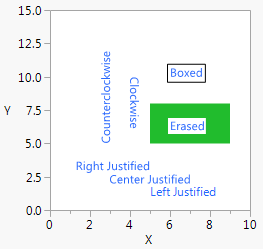Publication date: 11/29/2021

You can use Text() to draw text at a given location.

`Text( <properties>, ( {x, y}|{left, bottom, right, top} ), "text" );`

The point and text can be in any order and repeated. You can precede the point and text with an optional first argument: Center Justified, Right Justified, Erased, Boxed, Counterclockwise, or Clockwise. Erased is for removing whatever would otherwise obscure the text in a graph. It paints a background-colored rectangle behind the text.

In the following example, notice how the erased text appears inside a white box over the green Rect().

`mytext = New Window( "Adding Text",`
`	Graph Box(`
`		Frame Size( 200, 200 ),`
`		Y Scale( 0, 15 ),`
`		X Scale( 0, 10 ),`
`		Text Size( 9 );`
`		Text Color( "blue" );`
`		Text( {5, 1}, "Left Justified" );`
`		Text( Center Justified, {5, 2}, "Center Justified" );`
`		Text( Right Justified, {5, 3}, "Right Justified" );`
`		Fill Color( 4 );`
`		Rect( 5, 8, 9, 5, 1 );`
`		Text( Erased, {6, 6}, "Erased" );`
`		Text( Boxed, {6, 10}, "Boxed" );`
`		Text( Clockwise, {4, 10}, "Clockwise" );`
`		Text( Counterclockwise, {3, 5}, "Counterclockwise" );`
`	)`
`);`

Figure 12.19 Drawing Text in a Graph BoxThere is a variant of the Text() function that draws a string inside the rectangle that is specified by four coordinates. Here is the syntax:

`Text( {left, top, right, bottom}, string);`